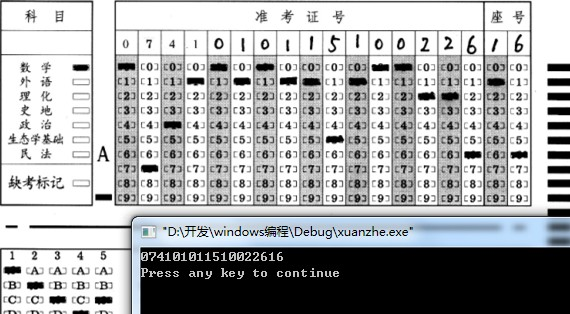#0
0
01. 云栖社区>
2. 博客>
3. 正文

## 网上阅卷系统自动识别功能代码/*

功能：网上阅卷系统的识别
作者：ma6174
邮箱：ma6174@163.com
时间：2012年2月23日
其他：包含的头文件<graphics.h>来则easyx的图形库
*/
#include<stdio.h>
#include<graphics.h>
#include<stdlib.h>
#include<string.h>
#include<conio.h>
#include<windows.h>
//定义方格的大小和方格的间距，根据实际情况做相应的修改
#define BOX_X 42
#define BOX_Y 13
#define BLANK_X 18
#define BLANK_Y 25
//颜色分量类
class rgb
{
public:
BYTE red;
BYTE green;
BYTE blue;
};
//判断一个点是不是黑色
bool judge_black(rgb color)
{
if(color.blue+color.green+color.red<30)//可能会有点误差，根据实际情况调整
return true;//黑色，有标记
return false;
}
//判断一个方格有没有被填涂
bool judge_box(int x,int y,HDC hdc)
{
int i,j,total=0,count=0;
COLORREF color;
for(i=x+2;i<x+BOX_X-2;i++)//循环判断所有点
{
for(j=y+1;j<y+BOX_Y-1;j++)
{
total++;
color=GetPixel(hdc,i,j);
rgb temp;
temp.red=GetRValue(color);
temp.green=GetGValue(color);
temp.blue=GetBValue(color);
if(judge_black(temp)==true)
count++;
}
}
if(double(count)/total>0.5)//黑色的比例大于50%则认为该方格被填涂
return true;
return false;
}
//判断所有的，入口参数分别是起始横坐标，起始纵坐标，行数，列数，窗口句柄
void judge_all(int start_x,int start_y,int hangshu,int lieshu,HDC hdc)
{
int i,j;
for(i=0;i<hangshu;i++)
{
for(j=0;j<lieshu;j++)
{
//            Sleep(50);
//            circle(start_x+i*(BOX_X+BLANK_X),start_y+j*(BOX_Y+BLANK_Y),10);
if(judge_box(start_x+i*(BOX_X+BLANK_X),start_y+j*(BOX_Y+BLANK_Y),hdc)==true)
{
printf("%d",j);
//                outtextxy(start_x+i*(BOX_X+BLANK_X),start_y+j*(BOX_Y+BLANK_Y),"A");
}
}
//        printf("\n");
}
}
int main()
{
int start_x,start_y,total=0,count=0;
initgraph(1440,900);
cleardevice();
IMAGE img;
HDC hdc = GetImageHDC();
MOUSEMSG m;
while(1)//通过鼠标点击得到起始位置
{
m=GetMouseMsg();
if(m.uMsg==WM_LBUTTONDOWN)
{
start_x=m.x;
start_y=m.y;
break;
}
}
judge_all(start_x,start_y,18,10,hdc);
printf("\n");
getch();
}

+ 关注